# Kerala SSLC Maths Model Question Paper 4 English Medium

Students can Download Kerala SSLC Maths Model Question Paper 4 English Medium, Kerala SSLC Maths Model Question Papers helps you to revise the complete Kerala State Syllabus and score more marks in your examinations.

## Kerala SSLC Maths Model Question Paper 4 English Medium

Time: 2½ Hours
Total Score: 80 Marks

Instructions

• Give explanations wherever necessary.
• First 15 minutes is Cool-off time. You may use the time to read the questions and plan your answers.
• No need to simplify irrationals like √2, √3, π etc., using approximations unless you are asked to do so.

Answer any three questions from 1 to 4. Each question carries 2 scores. (3 × 2 = 6)

Question 1.
In quadrilateral ABCD, ∠A = 100°, ∠B = 110°, ∠C = 50°
a) Where is the position of B?
b) Where is the position of D?a) Inside the circle
b) ∠D = 360 – 270 = 90°
On the circle.

Question 2.
In an arithmetic sequence, the 6th term is 14 and 14th term is 6.
a) Find a common difference.
b) Find the 20th term.
a) d = $$\frac{6-14}{14-6}$$ = $$\frac{-8}{8}$$ = 1
b) t20 = t14 + 6d = 6 + 6 × -1 = 6 – 6 = 0

Question 3.
First, five odd numbers which are multiples of five are given
a) Find mean.
b) Find the median.
5, 15, 25, 35, 45
$$\frac{5+15+25+35+45}{5}=\frac{125}{5}=25$$
b) Median = 25

Question 4.
In a box, there are pieces of paper in which the digits 1.5 are written. If we take a paper without looking.
a) Probability of being odd is.
b) What is the probability of being prime?
1, 2, 3, 4, 5
a) 3/5
b) 3/5

Answer any 5 questions from 5 to 11. Each question carries 3 marks each. (5 × 3 = 15)

Question 5.
Consider the arithmetic sequence $$\frac{17}{7}, \frac{31}{7}, \frac{45}{7}, \ldots \ldots$$
a) Write the algebric form of this sequence.
b) Find wheather.natural numbers are a term of this sequence.
17, 31, 45, ……….
a) 14n + 3/7
b) No
Reason = $$\frac{14 n+3}{7}=2 n+3 / 7$$

Question 6.
When a circle of radius 13 unit and mid-point (4, 5) is drawn, the point A and B cuts the x-axis.
a) Find the coordinates of A and B
b) Find the length of AB.a) (x – 4)2 + 52 = 132
(x – 4)2 = 169 – 25
(x – 4)2 = 144
x – 4 = 12, x – 4 = -12
x = 8, x = -8
A (8, 0), B (-8, 0)
b) AB = 16

Question 7.
a) Draw a circle of radius 3 cm
b) Draw a triangle with angles 55° and 75°, and the abovecirle as the incircle of the triangle.Question 8.
A chord AB is drawn 4 cm away from the centre of a circle. Chord makes an angle of 120° at the centre.
a) Draw a rough figure.
b) Find the radius of the circle.
c) Find the length of AB.Question 9.
In polynomial x2 – 3x
a) What should be added with this polynomial for (x – 2) to be a factor.
b) If (x – 1) is a factor of x2 – kx + 2. What is the value of k?
a) P(2) = 22 – 3 × 2 = 4 – 6 = -2
P(2) = 0
(To make P(2) = 0 add 2)
b) x2 – kx + 2 if x – 1 is a factor
P(1) = 0
⇒ 1 – k ×1 + 2 = 0
⇒ 3 – k = 0
⇒ k = 3

Question 10.
In the picture, a rhombus is drawn joining the mid-points of a rectangle and another rectangle is drawn joining the mid-points of the rhombus and it is shaded. If we put a dot on the figure without looking at what is the probability that it is inside the shaded part.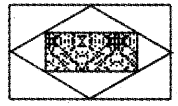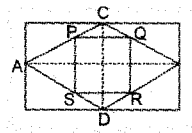PQ = ½ AB
SR = ½ AB
PS = ½ CD
QR = ½ CD
Area of PQSR = PQ × QR
= ½ AB × ½ CD
= $$\frac { 1 }{ 4 }$$ (AB × CD)
= $$\frac { 1 }{ 4 }$$ × (area of large rectangle)
Probability = $$\frac { 1 }{ 4 }$$ or $$\frac{4}{16}=\frac{1}{4}$$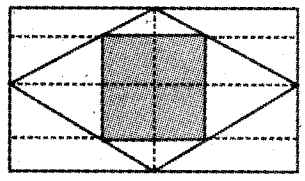Question 11.
If the first term of the arithmetic sequence is x and the common difference is 3.
a) What is the next term?
b) Write arithmetic sequences in which the product of the first two terms is 28, and the common difference is 3.
First term = x
a) Second term = x + 3
b) x (x + 3) = 28
x2 + 3x = 28
x2 + 3x – 28 = 0
(x + 7) (x – 4) = 0
x = -7, 4
Sequence = -7, -4, -3 … or 4, 7, 10

Answer any 7 questions from 12 to 21. Each question carries 4 score. (7 × 4 = 28)

Question 12.
2x + 1, 4x + 2, 6x + 3
a) If this is an arithmetic sequence what is the common difference?
b) What is the 10th term?
c) If the 10th term is 70. Find the value of x.
a) d = 4x + 2 – (2x + 1) = 2x + 1
b) t10 = 2x + 1 + 9 (2x + 1) = 20x + 10
c) 20x + 10 = 70
20x = 60
x = 60/20 = 3

Question 13.
In the figure ABCD is a cyclic quadrilateral PQ, R are the tangents of points at A, C.
∠BAQ = 50°, ∠ABD = 60°, ∠BCR = 30°.
Find the following angles.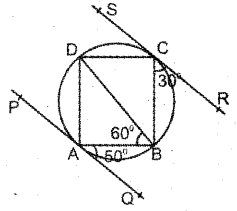b) ∠CDB
c) ∠BCD
d) ∠DCS
b) ∠CDB = 30°
c) ∠BCD = 110°
d) ∠DCS = ∠DBC = 40°

Question 14.
The distance from the centre to a point outside the circle of radius is 3 units larger than 2 times its radius. The length of the tangent is 1 unit less than the distance between the centre and external point.
a) If the radius is x. Find the distance between the centre and external point and length at a tangent.
b) Find its length using a second-degree equation.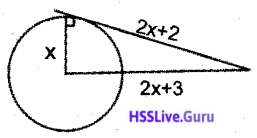a) 2x + 3
2x + 3 – 1 = 2x + 2
b) (2x + 3)2 = (2x + 2)2 + x2
⇒ 4x2 + 12x + 9 = 4x2 + 8x + 4 + x2
⇒ x2 + 8x – 12x + 4 – 9 = 0
⇒ x2 – 4x -5 = 0
⇒ (x – 5)(x + 1) = 0
⇒ x = 5, -1
∴ r = 5, d = 13, l = 12

Question 15.
The angle between lateral edge and base diagonal of a square pyramid is 30°. If the length of lateral edge is 20 cm.
a) Find the height of the square pyramid.
b) Find the length of diagonal.
c) Find the length of base edge.
d) Find slant height.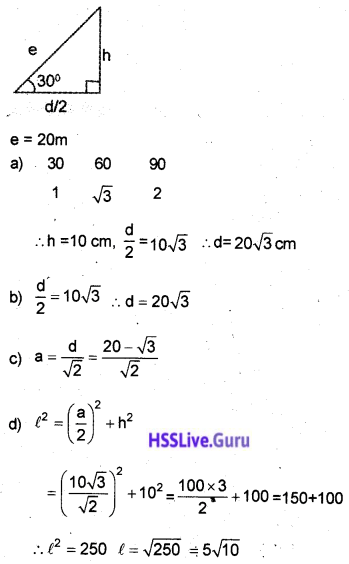Question 16.
If x2 – 1 is a factor of P(x) = ax3 + bx2 + cx + d
a) Write two first degree factors of P(x)
b) Prove a + c = b + d
a) (x + 1), (x – 1)
b) P(1) = 0, P(-1) = 0
If P(-1) = 0 P(-1) = 0
⇒ – a × (-1)3 + b × (-1)2 + c × -1 + d = 0
⇒ -a + b – c + d = 0
⇒ a + c = b + d

Question 17.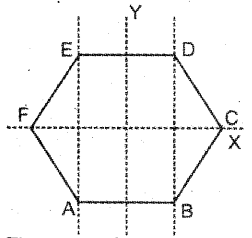The centre of a regular hexagon is the origin with sides 4 cm.
Write the co-ordinates of all its vertices.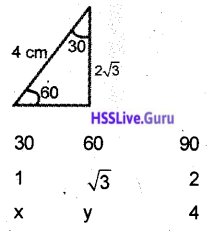x = 2, y = 2√3
E = (-2, 2√3)
D = (2, 2√3)
C = (4, 0)
B = (2, -2√3)
A = (-2, -2√3)
F = (-4, 0)

Question 18.
The radius of a vessel of cone shape is 6 cm and height 18 cm. It is filled with water. The radius of another cone-shaped vessel is 27 cm and height 24 cm.
a) What is the volume of the first cone?
b) Find the volume of the larger cone.
c) If we pour the water from the small cone to the larger one by holding it upside down. What will be the height of the water level?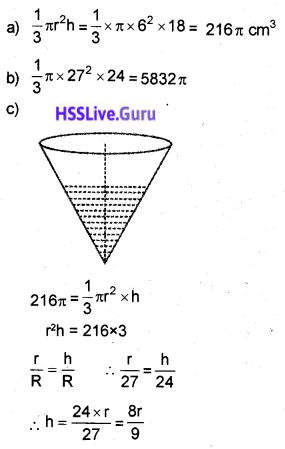Question 19.
The number of students in the 10th standard is arranged in a table according to their height.

 Height (cm) No. of Students 130 – 135 2 135 – 140 5 140 – 145 8 145 – 150 10 150 – 155 7 155 – 160 6 160 – 165 3

a) Find the height of the student in 16th place, if all are arranged according to their heights in order.
b) Which student’s height comes as a median.
c) Find the median height.
Less than 135 – 2
Less than 140 – 7
Less than 145 – 15
Less than 150 – 25
Less than 155 – 32
Less than 160 – 38
Less than 165 – 41
N = 41
N/2 = 20.5b) 21st
c) 145.25 + 5 × 0.5 = 145.25 + 2.5 = 147.75

Question 20.
a) Draw a rectangle with sides 3 cm, 5 cm.
b) Draw a square with one sides √15 cm and the same area that of the rectangle.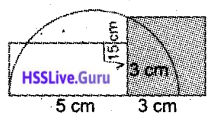Question 21.
The line joining A(1, 3), B(5, 2) and C(1, 1), D (5, 4) intersects at P.
a) Write the equation of the line AB.
b) Write the equation of the line CD
c) Find the coordinates of P.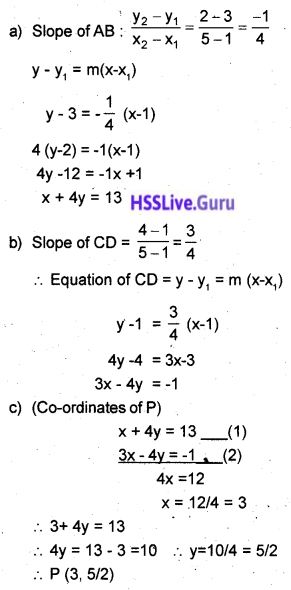Write any 5 questions from 22 to 28. Each question carries 5 marks. (5 × 5 = 25)

Question 22.
In the figure a model of aframe which hangs a weight is given BC = 5 unit, BE = 2 unit, AE = 6 unit. ∠BDC = 35°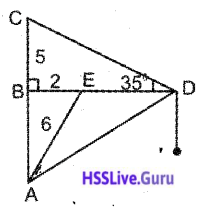a) Find the length of AB.
b) Find the length of CD
c) Find the length of EDQuestion 23.
Consider the following arithmetic sequences
8, 14, 20, 26, …………
34, 38, 42, 46, ………….
a) Write the common difference of the above two sequences.
b) Write the algebraic form of two sequences.
c) Check whether is there any common term at the same position for these two series.
a) 6, 4
b) 6n + 2
2n + 30
c) 6n + 2 = 4n + 30
2n = 28
n = 14
(14th term will be equal)
6 × 14 + 2 = 84 + 2 = 86
Equal term = 86

Question 24.
There are two taps for a tank. One is to fill and the other is to empty. The second tap takes 5 minutes more to empty the full tanks than the first tap to fill the tank. If two were open it took 1 hr to fill the tank.
a) If the first tap takes x minutes to fill the tank. What is the time taken to empty the tank by the second tap?
b) From a quadratic equation by taking the volume fill in the tank in a minute,
c) Find the time taken by the two taps.
a) x + 5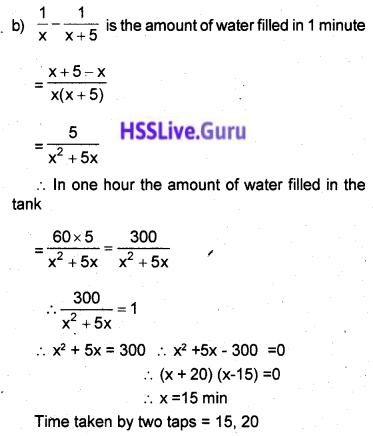Question 25.
A solid hemisphere is attached at one end of a solid core. Height of the cone is equal to the diameter of the hemisphere. This solid figure is melted and recast into a sphere of radius equal to $$\frac{1}{3}^{\mathrm{rd}}$$ of the diameter of the hemisphere.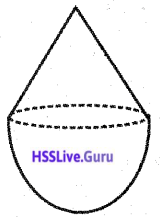a) If the radius of the hemisphere is ‘r’. What is the height of the cone?
b) Find the volume of the solid shape.
c) What is the radius of the sphere?
d) Find the volume of the sphere.
e) How many such spheres can be made from the solid shape?
a) 2r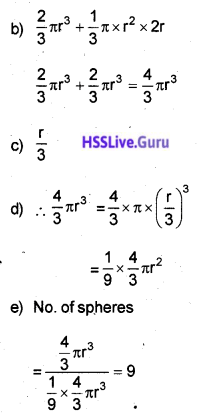Question 26.
Two lines 2x – 3y + 7 = 0, 3x + 2y – 9 = 0 intersects at P.
a) Find the co-ordinates of P.
b) Find the equation of the line which passes through P and has a slope ½.
c) Prove that the two lines 2x – 3y + 7 = 0 and 3x + 2y – 9 = 0 are perpendicular.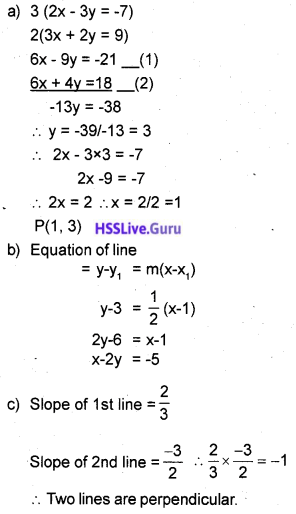Question 27.
CQ and CS are tangent from C to the outer circle of ∆ABC. I is the centre of the incircle and J is the centre of the outer circle.a) Prove that the perimeter of ∆ABC is equal to CQ + CS.
b) If CP = 5 cm, CQ = 8 cm, IP = 2 cm. Find the radius of the outer circle.
Perimeter of ∆ABC = CB + BR + AR + AC
= (CB + BQ) + (AS + AC)
= CQ + CS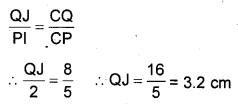Question 28.
a) Draw a square of side 5 cm.
b) Draw the incircle and circumcircle of the square.
c) Find the ratio of the incircle and circumcircle of the square.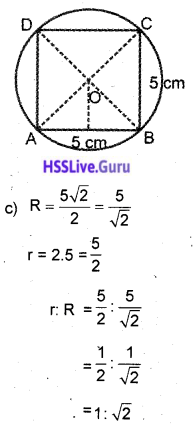Read the mathematical concept carefully and answer the following question. (6 × 1 = 6)

Question 29.
We can draw an incircle and circumcircle to a triangle. What about in the case of quadrilaterals….?. Can we draw both to a quadrilateral…?